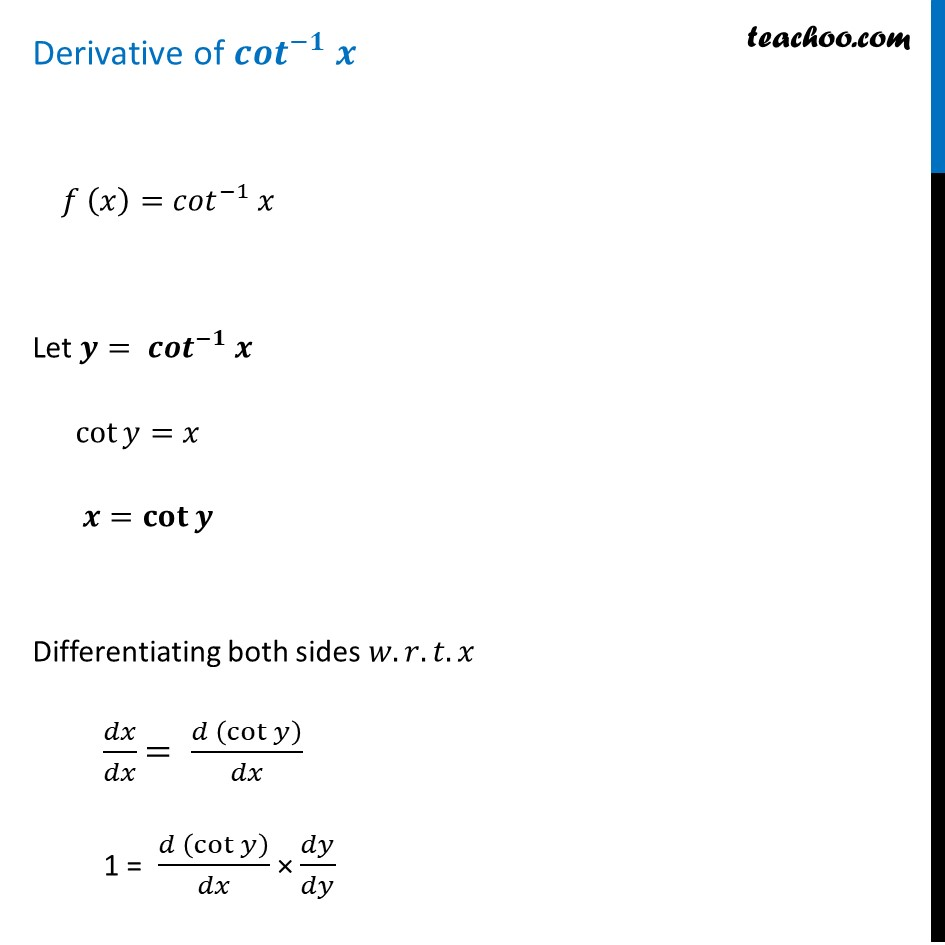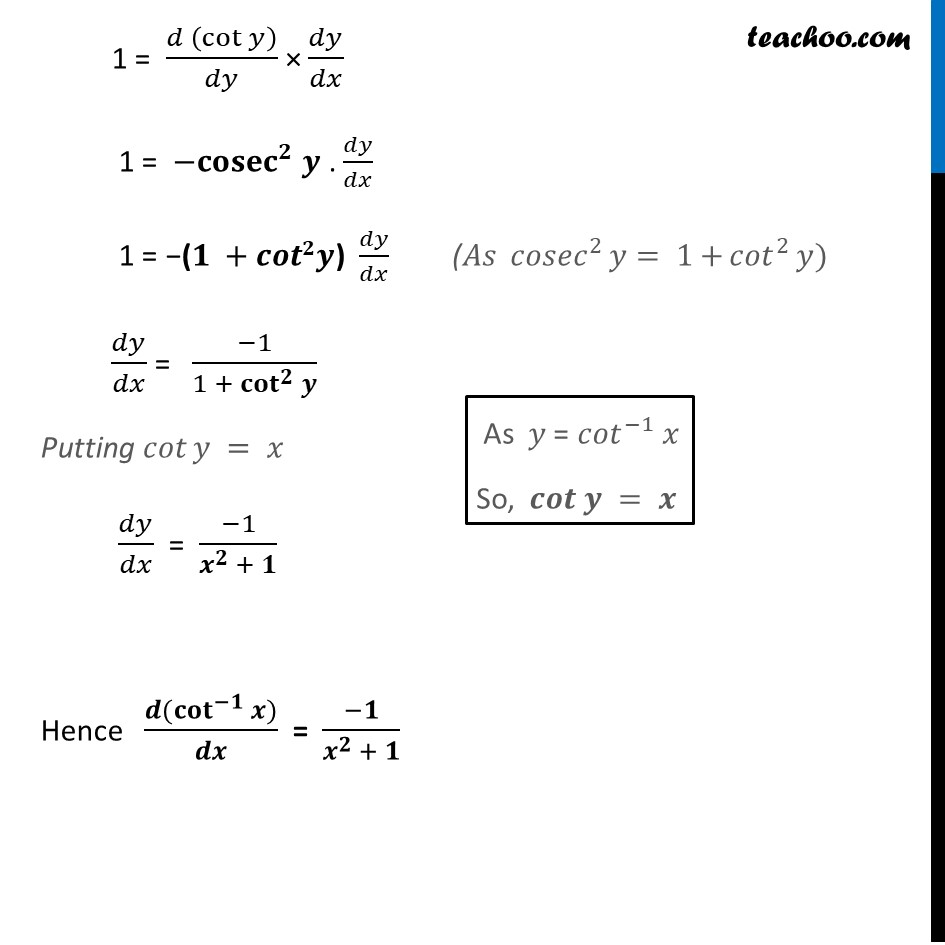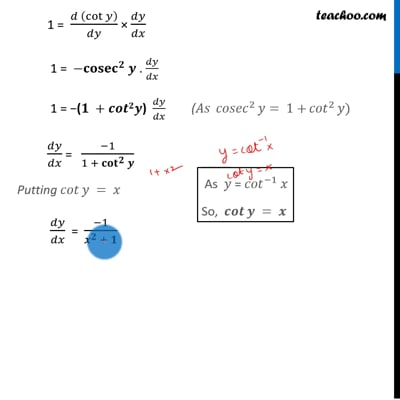Finding derivative of Inverse trigonometric functions

Chapter 5 Class 12 Continuity and Differentiability
Concept wiseThis video is only available for Teachoo black users

Introducing your new favourite teacher - Teachoo Black, at only ₹83 per month

### Transcript

Derivative of 〖𝒄𝒐𝒕〗^(−𝟏) 𝒙 𝑓 (𝑥)=〖𝑐𝑜𝑡〗^(−1) 𝑥 Let 𝒚= 〖𝒄𝒐𝒕〗^(−𝟏) 𝒙 cot⁡〖𝑦=𝑥〗 𝒙=𝐜𝐨𝐭⁡〖𝒚 〗 Differentiating both sides 𝑤.𝑟.𝑡.𝑥 𝑑𝑥/𝑑𝑥 = (𝑑 (cot⁡𝑦 ))/𝑑𝑥 1 = (𝑑 (cot⁡𝑦 ))/𝑑𝑥 × 𝑑𝑦/𝑑𝑦 1 = (𝑑 (cot⁡𝑦 ))/𝑑𝑦 × 𝑑𝑦/𝑑𝑥 1 = −𝐜𝐨〖𝐬𝐞𝐜〗^𝟐 𝒚 . 𝑑𝑦/𝑑𝑥 1 = −(𝟏 +𝒄𝒐𝒕𝟐𝒚) 𝑑𝑦/𝑑𝑥 𝑑𝑦/𝑑𝑥 = (−1)/(1 + 〖𝐜𝐨𝐭〗^𝟐⁡𝒚 ) Putting 𝑐𝑜𝑡⁡𝑦 = 𝑥 𝑑𝑦/𝑑𝑥 = (−1)/(𝒙^𝟐 + 𝟏) Hence (𝒅(〖𝐜𝐨𝐭〗^(−𝟏)⁡〖𝒙)〗)/𝒅𝒙 = (−𝟏)/(𝒙^𝟐 + 𝟏) (𝐴𝑠 〖 𝑐𝑜𝑠𝑒𝑐〗^2⁡〖𝑦= 〖1+〗⁡〖𝑐𝑜𝑡〗^2⁡𝑦 〗) As 𝑦 = 〖𝑐𝑜𝑡〗^(−1) 𝑥 So, 𝒄𝒐𝒕⁡𝒚 = 𝒙